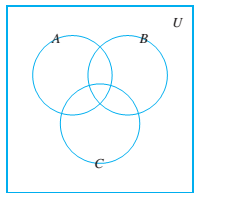# Consider the Venn diagram below. Illustrate one of the distributive laws by shading in the region corresponding to A ∪ ( B ∩ C ) on one copy of the diagram and ( A ∪ B ) ∩ ( A ∪ C ) on another. Illustrate the other distributive law by shading in the region corresponding to A ∪ ( B ∩ C ) on one copy of the diagram and ( A ∪ B ) ∩ ( A ∪ C ) on another. Illustrate one of De Morgan's laws by shading In the region corresponding to ( A ∪ B ) c on one copy of the diagram and A c ∩ B c on the other. (Leave the set C out of your diagrams.) Illustrate the other De Morgan's law by shad- ing in the region corresponding to on A c ∩ B c one copy of the diagram and A c ∩ B c on the other. (Leave the set C out of your diagrams.)### Discrete Mathematics With Applicat...

5th Edition
EPP + 1 other
Publisher: Cengage Learning,
ISBN: 9781337694193

#### Solutions

Chapter
Section### Discrete Mathematics With Applicat...

5th Edition
EPP + 1 other
Publisher: Cengage Learning,
ISBN: 9781337694193
Chapter 6.2, Problem 26ES
Textbook Problem
4 views

## Consider the Venn diagram below.Illustrate one of the distributive laws by shading in the region corresponding to   A ∪ ( B ∩ C ) on one copy of the diagram and   ( A ∪ B ) ∩ ( A ∪ C ) on another. Illustrate the other distributive law by shading in the region corresponding to A ∪ ( B ∩ C ) on one copy of the diagram and   ( A ∪ B ) ∩ ( A ∪ C ) on another. Illustrate one of De Morgan's laws by shading In the region corresponding to ( A ∪ B ) c on one copy of the diagram and A c ∩ B c on the other. (Leave the set C out of your diagrams.) Illustrate the other De Morgan's law by shad- ing in the region corresponding to on A c ∩ B c one copy of the diagram and A c ∩ B c on the other. (Leave the set C out of your diagrams.)

To determine

To Prove:

For all sets A,B,and C,

(AC)(BC)(AB)=ϕ

### Explanation of Solution

Given information:

Let  A , B and C be any set

Concept used:

:Union of sets:Intersection of sets

: subset of set

Calculation:

The objective is to prove that (AC)(BC)(AB)=ϕ, for all sets A,B,and C.

Consider,

(AC)(BC)(AB)={x|xACand xBCand xAB }={x|xACcand xBCcand xABc}By set difference law.={x|(xAand xC)and(xBand xC)and (xAandxB)}

={x|xAand (xB<

### Still sussing out bartleby?

Check out a sample textbook solution.

See a sample solution

#### The Solution to Your Study Problems

Bartleby provides explanations to thousands of textbook problems written by our experts, many with advanced degrees!

Get Started

Find more solutions based on key concepts
In Exercises 1-18, construct a truth table for each compound proposition. (pq)(~p)

Finite Mathematics for the Managerial, Life, and Social Sciences

Add the following signed number: (+7)+(8)

Elementary Technical Mathematics

Reminder Round all answers to two decimal places unless otherwise indicated. Finding an Equation of ChangeThe d...

Functions and Change: A Modeling Approach to College Algebra (MindTap Course List)

For f(x) = 6x4, f′(x) = _____. 24x 6x3 10x3 24x3

Study Guide for Stewart's Single Variable Calculus: Early Transcendentals, 8th

Distance Between Two Cargo Ships Ship A leaves port sailing north at a speed of 25 mph. A half hour later, Ship...

Applied Calculus for the Managerial, Life, and Social Sciences: A Brief Approach

In Exercises 516, evaluate the given quantity. log100,000

Finite Mathematics and Applied Calculus (MindTap Course List)

WRITING ABOUT CONCEPTS LHpitals Rule State LHpitals Rule.

Calculus: Early Transcendental Functions (MindTap Course List)